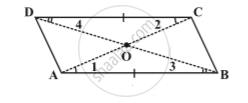Share

# Kinds of Quadrilaterals - Diagonals of a Parallelogram

#### text

Property: The diagonals of a parallelogram bisect each other .Given : ABCD is parallelogram , AC & BD intersect at O.
To prove : OA = OC and OB = OD .
Proof : In ∆ AOB and  ∆ COD , we know that
∠OAB = ∠ OCD   (Alternate angle )
∠OBA = ∠ ODC   (Alternate angle )
bar (AB) = bar (CD)  (opposite sides are equal in parallelogram)
∆ AOB ≅ ∆ COD     (ASA Axiom)
Hence OA = OC and OB = OD

### Shaalaa.com

Property: The diagonals of a parallelogram bisect each other . [00:06:20]
S
0%

S